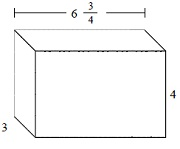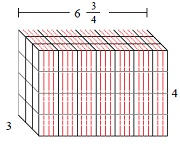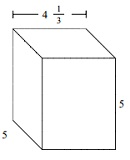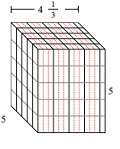# Volume of a Rectangular Prism with Fractional Edge Lengths

In this lesson, we find the volume of rectangular prisms with fractional edge lengths.

Formula for the volume of solid made of cubes with unit fractional edge lengths

l = number of cubes with unit fractional edge length along the length

w = number of cubes with unit fractional edge length along the width

h = number of cubes with unit fractional edge length along the height

k = unit fractional edge length

Volume of solid = l × k × w × k × h × k cubic units

Find the volume of following solid of cubes with unit fraction edge lengths. Each prisms unit is measured in cm (not to scale)### Solution

Step 1:

Solid of cubes with unit fraction edge lengthsStep 2:

Volume V = l w h = $6 \frac{3}{4} \times 3 \times 4$

= $9 \times \frac{3}{4} \times 4 \times \frac{3}{4} \times \frac{16}{3} \times \frac{3}{4}$

= 81 cu cm

Find the volume of following solid of cubes with unit fraction edge lengths. Each prisms unit is measured in cm (not to scale)### Solution

Step 1:

Solid of cubes with unit fraction edge lengthsStep 2:

Volume V = l w h = $4 \frac{1}{3} \times 5 \times 5$

= $13 \times \frac{1}{3} \times 15 \times \frac{1}{3} \times 15 \times \frac{1}{3}$

= $108 \frac{1}{3}$ cu cm